• ## VGGNet

2019-08-10 23:25:42
VGGNet
VGGNet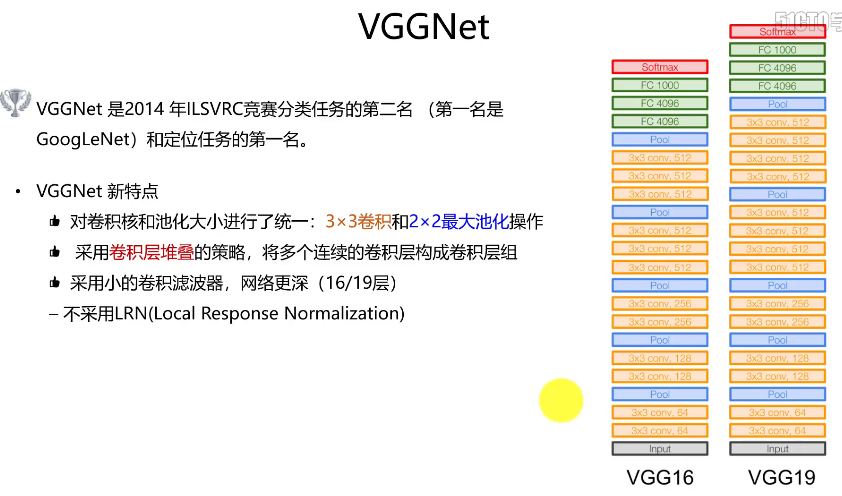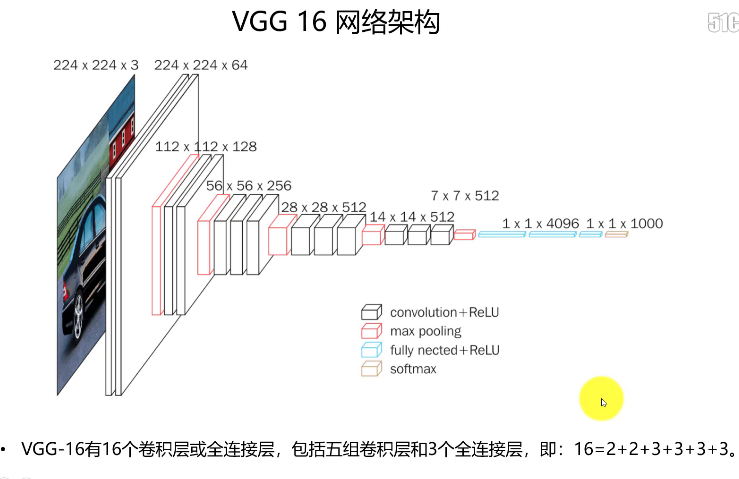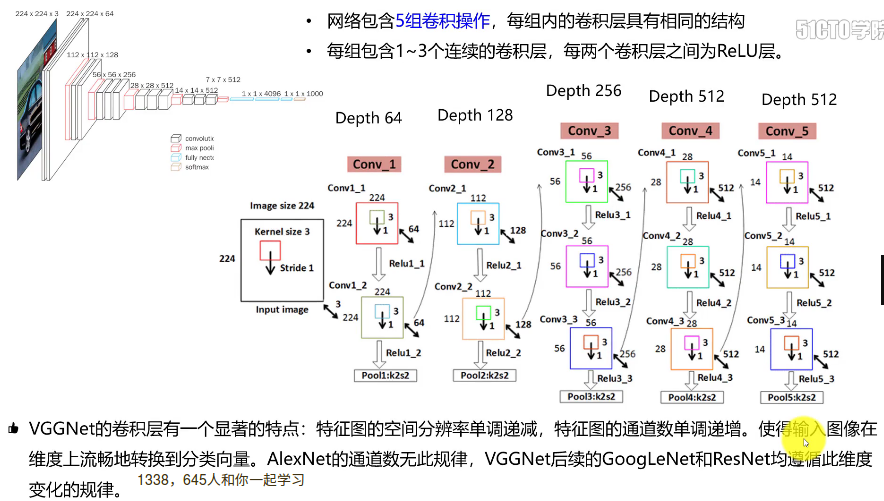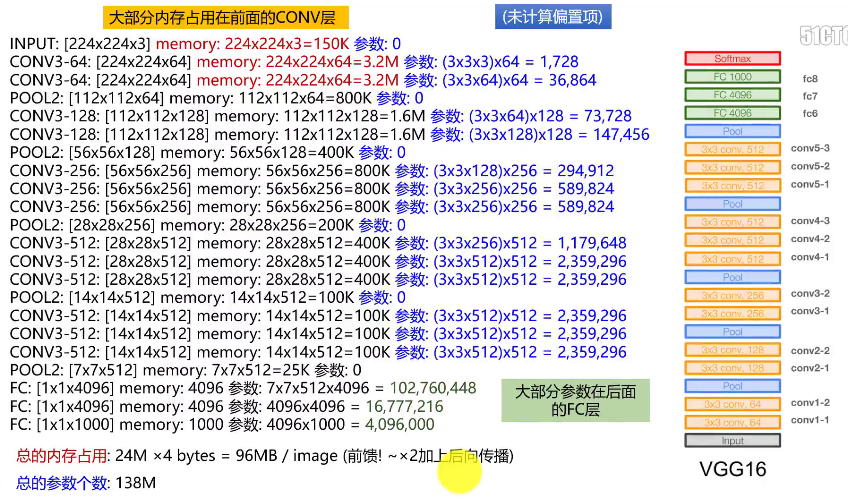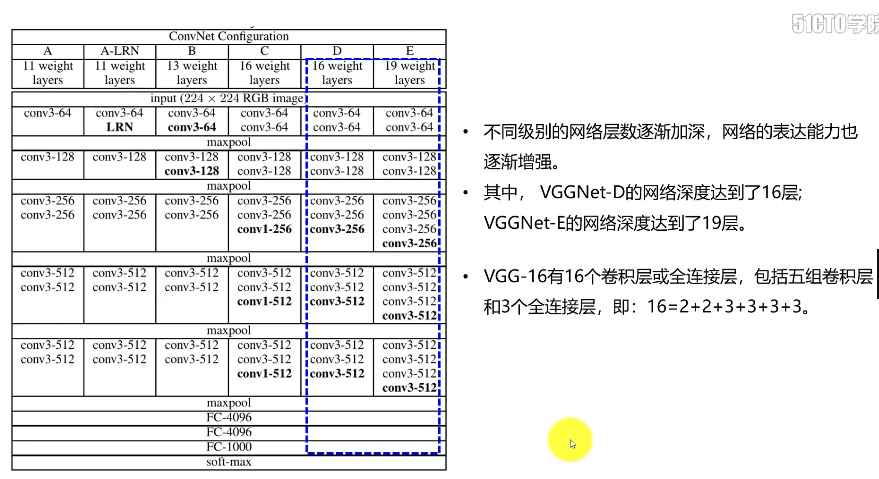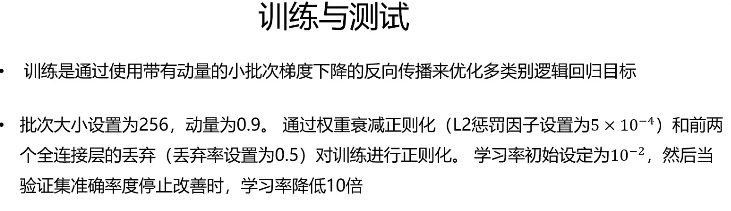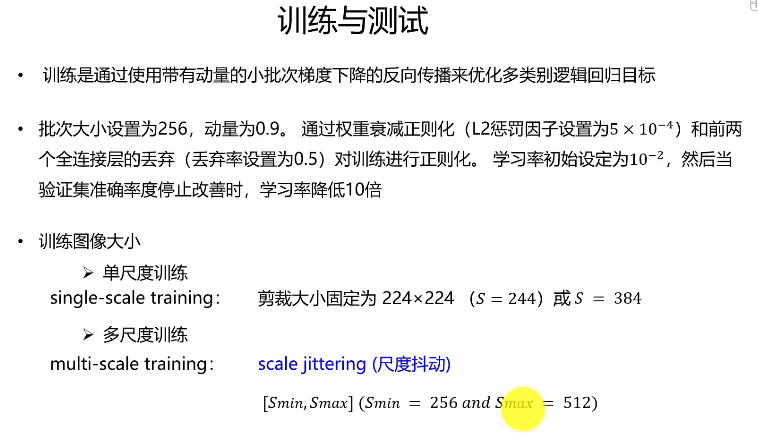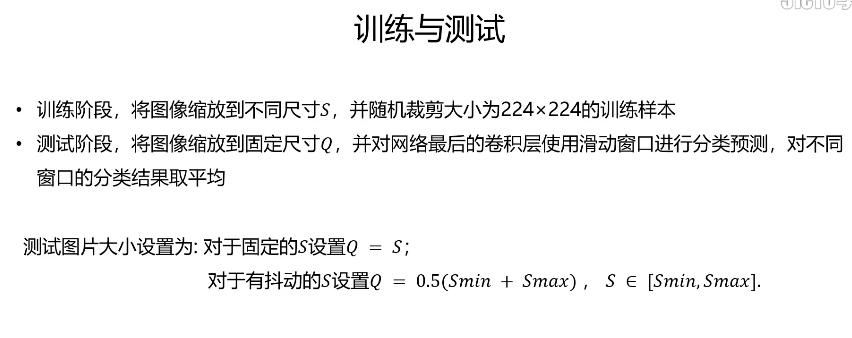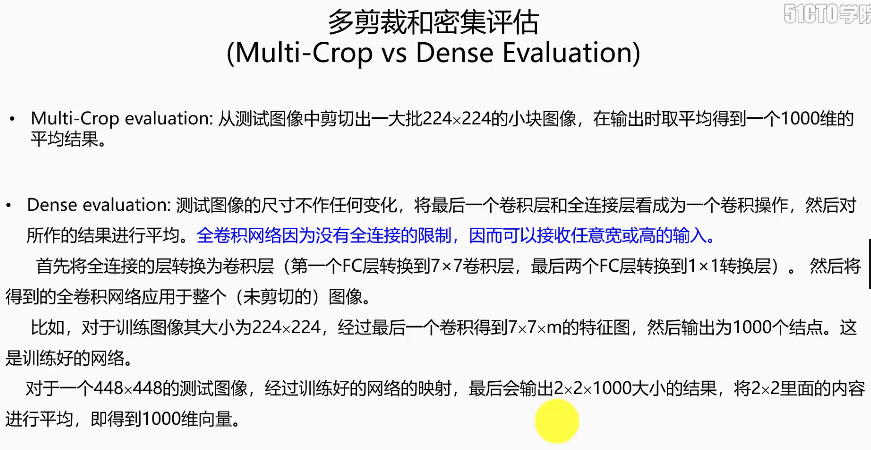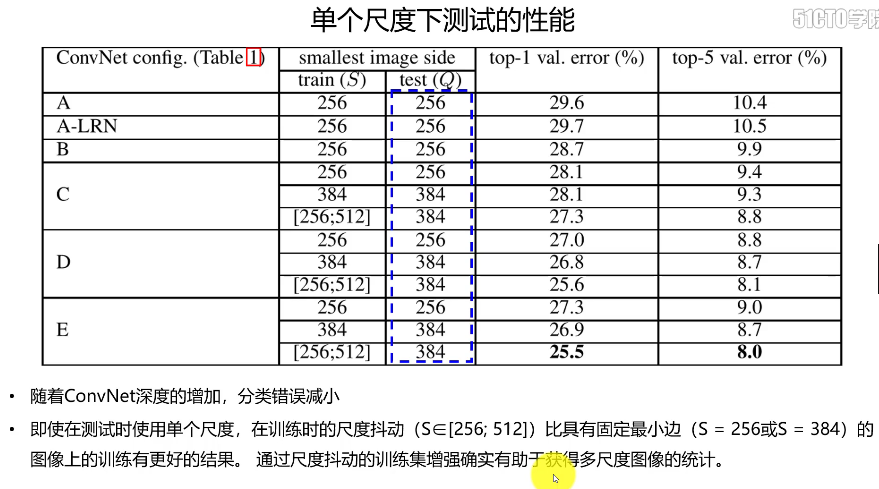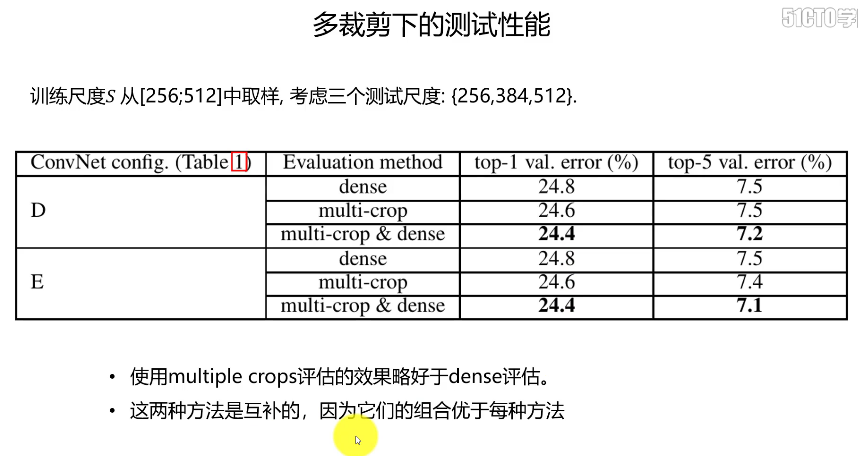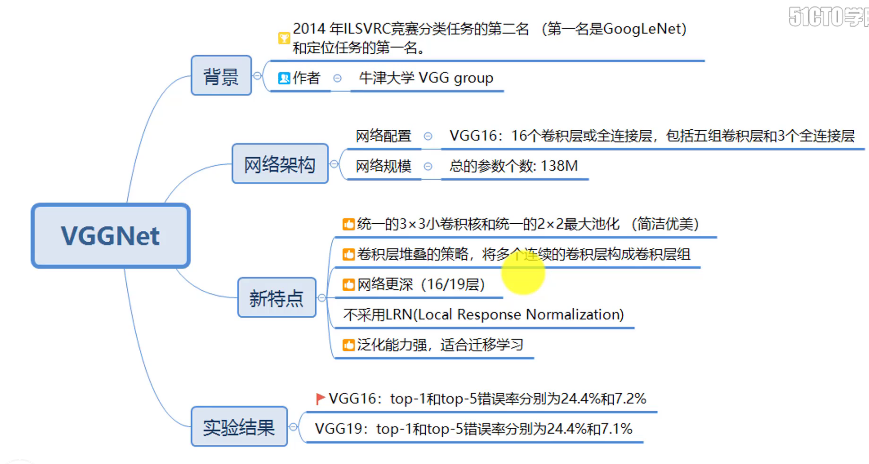展开全文深度学习
• ## VggNet

2020-02-12 09:23:31
VggNet是由牛津大学视觉几何组(Visual Geometry Group，VGG)的Karen Simonyan和Andrew Zisserman提出来的，VggNet在ILSVRC-2014中获得了定位任务的第一名和分类任务的第二名。 下面一起来看一下VggNet的论文《Very ...
VggNet是由牛津大学视觉几何组(Visual Geometry Group，VGG)的Karen Simonyan和Andrew Zisserman提出来的，VggNet在ILSVRC-2014中获得了定位任务的第一名和分类任务的第二名。

下面一起来看一下VggNet的论文《Very Deep Convolutional Networks for Large-Scale Image Recognition》。先直接给出网络设置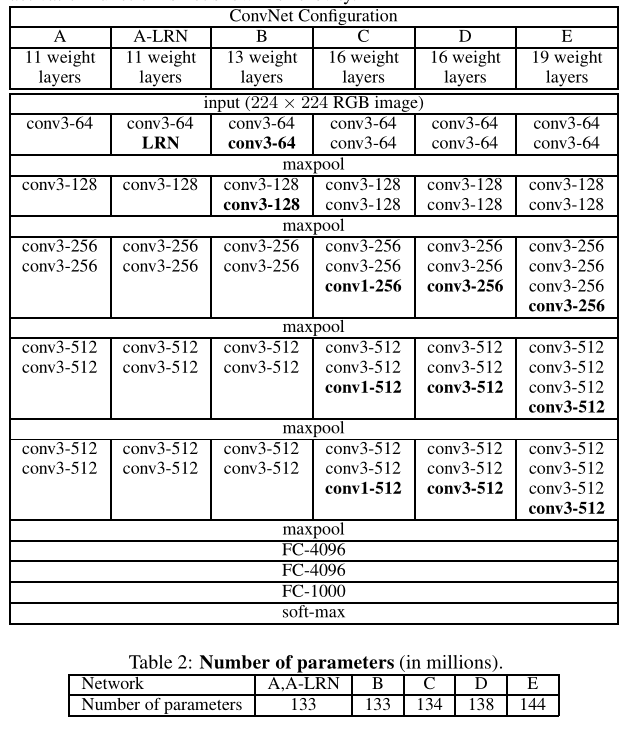这篇文章个人觉得最大的贡献就是用更小的卷积核实现更深的网络。

更小的卷积核的叠加可以实现大卷积核的感受野(receptive field)(两个3x3等价于1个5x5,3个3x3等价于一个7x7

同时减少了参数数量，比如说将3个3x3堆叠起来，如果输入和输出都有C个通道，则参数为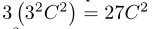而一个7x7的参数为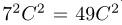可以看出参数有明显的减少

还有一个好处是由于层之间的ReLU函数，3个卷积层的堆叠的非线性效果也比1个卷积层的非线性效果要好

从网络设置可以看出，论文探讨了A-E 6种网络结构的性能，结果如下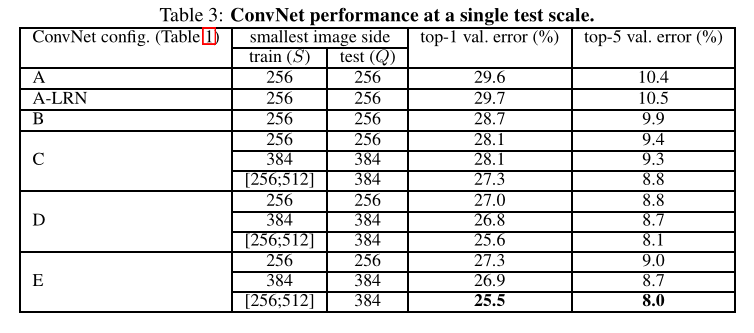即E结构获得了最好的性能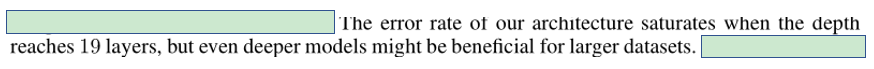同时，作者认为在更大的数据集上可能更深的网络结构会有更好的表现，即是说不同深度模型的表现与数据集的大小有关。

其他的论文提到的

图像预处理，包括random crop, horizon flip，RGB normalize

正则化，L2正则化，dropout

参数初始化，权重 w 从 N(0，0.01) 中采样，偏差 bias 初始化为0

单一尺度与多尺度训练

这里就不详细说了

展开全文• ## VGGnet

2020-07-18 22:15:23
VGGnet网络
一、简介
VGGnet分析的是卷积神经网络的深度和它所带来的性能之间的关系，构建了16-19层深的卷积神经网络（16层是VGG16,19层是VGG19),可以认为是用他卷积层的个数命名的网络，他成功的证明了卷积神经网络的深度可以影响到最终的网络性能，同时因为是卷积神经网络构成的，所以他的拓展性很强，迁移到其他的图片数据上的泛化能力也很好。
可以讲VGGnet理解为加深版本的alexnet，都是由卷积层和全连接层两大部分组成的。
二、特点

卷积核尺寸是3*3，这是因为捕捉上下左右，中心概念的最小尺寸
使用1*1的步长是在不影响输入输出的前提下，对输入的进行变化，在通过relu函数，提高决策函数的非线性。1x1的卷积神经网络可以来代替全连接层
使用3x3代替大范围卷积好处是可以来减少参数，相当于进行了多次的非线性映射，可以增加网络的拟合能力
通过减少卷积核大小并增加卷积数量使之达到同样的水平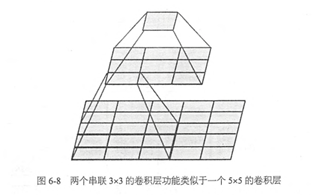三、网络结构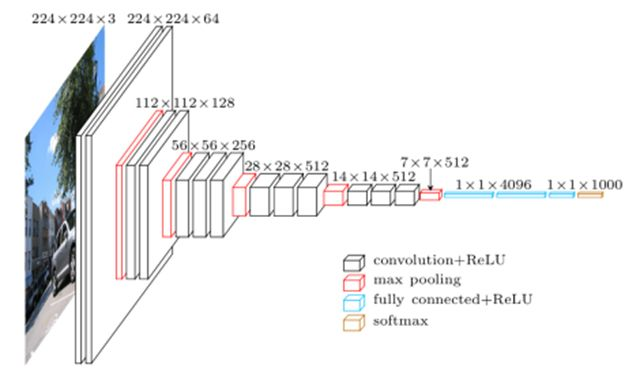首先在输入数据的大小上市224x224的RGB图像（也就是三通道的数据 x3），预处理计算每个通道的平均值，在减去。使得迭代更少，更快收敛。
图像经过每一层的网络的时候使用的都是3*3的卷积核，1步长，在有的卷积层里使用1x1的卷积核。
对于池化层使用的是2x2的卷积层，使用的步长是2。
卷积之后连接的是三个全连接层，前两个均是4096个通道，第三个全链接使用的是1000个通道，用来分类，所有全连接网络的配置是一样的。
所有隐藏层（每个conv层中间）都使用ReLU作为激活函数
四、THINK
1、选择采用33的卷积核是因为33是最小的能够捕捉像素8邻域信息的的尺寸。
2、使用1*1的卷积核目的是在不影响输入输出的维度情况下，对输入进行形变，再通过ReLU进行非线性处理，提高决策函数的非线性。
3、2个33卷积堆叠等于1个55卷积，3个3X3堆叠等于1个7*7卷积，感受野大小不变，而采用更多层、更小的卷积核可以引入更多非线性（更多的隐藏层，从而带来更多非线性函数），提高决策函数判决力，并且带来更少参数。
4、每个VGG网络都有3个FC层，5个池化层，1个softmax层。
5、在FC层中间采用dropout层，防止过拟合。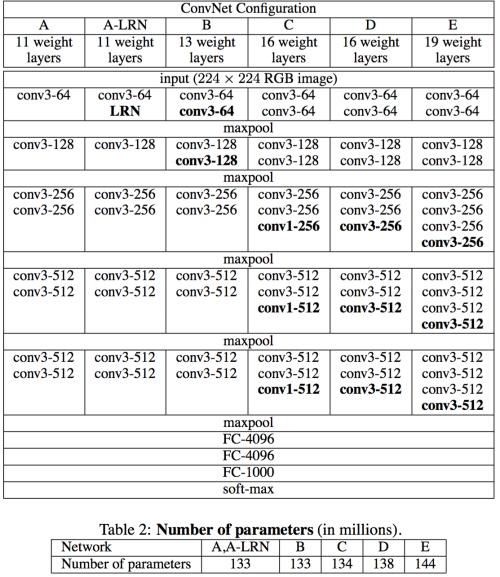五、训练
卷积层和全连接层的唯一区别就是卷积层的神经元和输入是局部联系的，并且同一个通道（channel）内的不同神经元共享权值（weight）。卷积层和全连接层的计算实际上相同，因此可以将全连接层转换为卷积层，只要将卷积核大小设置为输入空间大小即可：例如输入为77512，第一层全连接层输出4096；我们可以将其看作卷积核大小为77，步长为1，没有填充，输出为11*4096的卷积层。这样的好处在于输入图像的大小不再受限制，因此可以高效地对图像作滑动窗式预测；而且全连接层的计算量比较大，等效卷积层的计算量减小了，这样既达到了目的又十分高效。
六、Model
################################################################
# step 2 定义函数                                                #
################################################################
def weight_variable(shape):
return tf.Variable(tf.truncated_normal(shape,stddev=0.1))
def bias_variable(shape):
return tf.Variable(tf.constant(0.1,shape=shape))
def conv2d(x,w):
def max_pool_2x2(x):

################################################################
# step 3 定义容器                                                #
################################################################
x = tf.placeholder(tf.float32,[None,224,224,3])
y = tf.placeholder(tf.float32,[None,4])
keep_prob = tf.placeholder(tf.float32)

# 定义输入数据维度
x_image = tf.reshape(x,[-1,224,224,3])

################################################################
# step 4 定义一层                                                #
################################################################
w_con1 = weight_variable([3,3,3,64])
b_con1 = bias_variable()
conv_1 = tf.nn.relu(conv2d(x_image,w_con1)+b_con1)

w_con2 = weight_variable([3,3,64,64])
b_con2 = bias_variable()
conv_2 = tf.nn.relu(conv2d(conv_1,w_con2)+b_con2)

pool_1 = max_pool_2x2(conv_2)

# 输出为112*112*64
################################################################
# step 4 定义二层                                                #
################################################################
w_con3 = weight_variable([3,3,64,128])
b_con3 = bias_variable()
conv_3 = tf.nn.relu(conv2d(pool_1,w_con3)+b_con3)

w_con4 = weight_variable([3,3,128,128])
b_con4 = bias_variable()
conv_4 = tf.nn.relu(conv2d(conv_3,w_con4)+b_con4)

pool_2 = max_pool_2x2(conv_4)
# 输出为56*56*128

################################################################
# step 4 定义三层                                                #
################################################################
w_con5 = weight_variable([3,3,128,256])
b_con5 = bias_variable()
conv_5 = tf.nn.relu(conv2d(pool_2,w_con5)+b_con5)

w_con6 = weight_variable([3,3,256,256])
b_con6 = bias_variable()
conv_6 = tf.nn.relu(conv2d(conv_5,w_con6)+b_con6)

w_con7 = weight_variable([3,3,256,256])
b_con7 = bias_variable()
conv_7 = tf.nn.relu(conv2d(conv_6,w_con7)+b_con7)

w_con8 = weight_variable([3,3,256,256])
b_con8 = bias_variable()
conv_8 = tf.nn.relu(conv2d(conv_7,w_con8)+b_con8)

pool_3 = max_pool_2x2(conv_8)
# 输出为28*28*256

################################################################
# step 4 定义四层                                                #
################################################################
w_con9 = weight_variable([3,3,256,512])
b_con9 = bias_variable()
conv_9 = tf.nn.relu(conv2d(pool_3,w_con9)+b_con9)

w_con10 = weight_variable([3,3,512,512])
b_con10 = bias_variable()
conv_10 = tf.nn.relu(conv2d(conv_9,w_con10)+b_con10)

w_con11 = weight_variable([3,3,512,512])
b_con11 = bias_variable()
conv_11 = tf.nn.relu(conv2d(conv_10,w_con11)+b_con11)

w_con12 = weight_variable([3,3,512,512])
b_con12 = bias_variable()
conv_12 = tf.nn.relu(conv2d(conv_11,w_con12)+b_con12)

pool_4 = max_pool_2x2(conv_12)
# 输出为14*14*512

################################################################
# step 4 定义五层                                                #
################################################################
w_con13 = weight_variable([3,3,512,512])
b_con13 = bias_variable()
conv_13 = tf.nn.relu(conv2d(pool_4,w_con13)+b_con13)

w_con14 = weight_variable([3,3,512,512])
b_con14 = bias_variable()
conv_14 = tf.nn.relu(conv2d(conv_13,w_con14)+b_con14)

w_con15 = weight_variable([3,3,512,512])
b_con15 = bias_variable()
conv_15 = tf.nn.relu(conv2d(conv_14,w_con15)+b_con15)

w_con16 = weight_variable([3,3,512,512])
b_con16 = bias_variable()
conv_16 = tf.nn.relu(conv2d(conv_15,w_con16)+b_con16)

pool_5 = max_pool_2x2(conv_16)
# 输出为7*7*512

################################################################
# step 5 定义全连接                                              #
################################################################
# 扁平化
Pool_flat = tf.reshape(pool_5,[-1,7*7*512])

w_fuc_1 = weight_variable([7*7*512,4096])
b_fuc_1 = bias_variable()
h_fc1 = tf.nn.relu(tf.matmul(Pool_flat,w_fuc_1)+b_fuc_1)
#keep_prob用来表示神经元的输出概率
h_fc1_drop = tf.nn.dropout(h_fc1, keep_prob)  #进行dropout操作

w_fuc_2 = weight_variable([4096,4096])
b_fuc_2 = bias_variable()
h_fc2 = tf.nn.relu(tf.matmul(h_fc1_drop,w_fuc_2)+b_fuc_2)
#keep_prob用来表示神经元的输出概率
h_fc2_drop = tf.nn.dropout(h_fc2, keep_prob)  #进行dropout操作

w_fuc_3 = weight_variable([4096,1000])
b_fuc_3 = bias_variable()
h_fc3 =tf.matmul(h_fc2_drop,w_fuc_3)+b_fuc_3
h_fc3_drop = tf.nn.dropout(h_fc3, keep_prob)  #进行dropout操作

w_fuc_4 = weight_variable([1000,10])
b_fuc_4 = bias_variable()
h_fc4 =tf.matmul(h_fc3_drop,w_fuc_4)+b_fuc_4
# 计算输出
prediction = tf.nn.softmax(h_fc4)

致谢
https://www.cnblogs.com/gujiangtaoFuture/articles/12173705.html
https://www.cnblogs.com/aiblbns/p/11124656.html
https://www.cnblogs.com/mtcnn/p/9411743.html


展开全文tensorflow 神经网络 深度学习
• ## VGGNet

2019-06-20 11:38:11
转自：https://blog.csdn.net/loveliuzz/article/details/79080194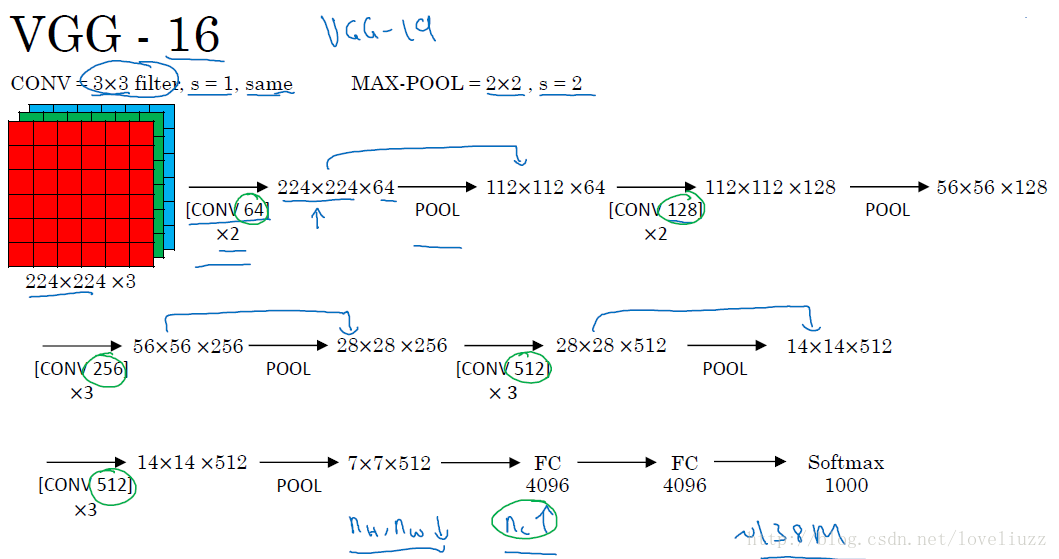VGGNet探索了卷积神经网络的深度与其性能之间的关系，通过反复堆叠3×3的小型卷积核和2×2的最大池化层
VGGNet成功地构筑了16~19层深的卷积神经网络。VGGNet相比之前state-of-the-art的网络结构，错误率大幅下降
VGGNet论文中全部使用了3×3的小型卷积核和2×2的最大池化核，通过不断加深网络结构来提升性能。
VGG-16和VGG-19结构如下：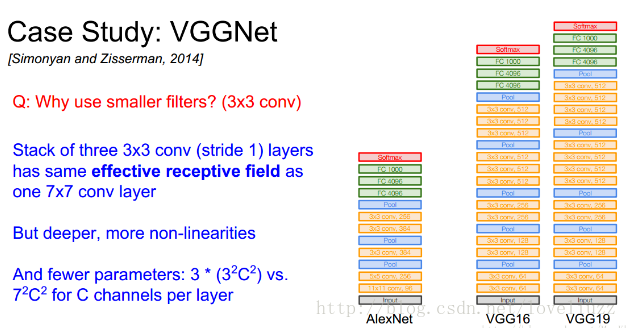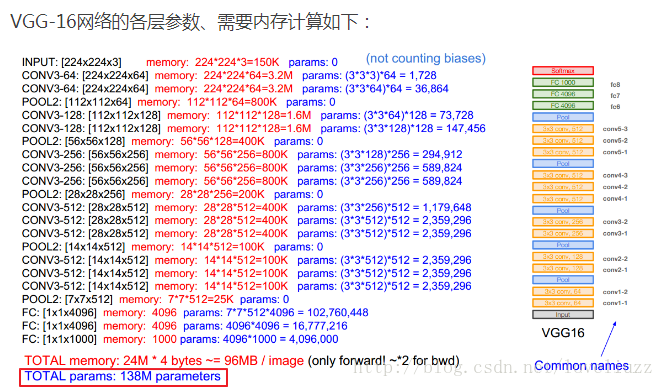总结：
（1）VGG-16网络中的16代表的含义为：含有参数的有16个层，共包含参数约为1.38亿。
（2）VGG-16网络结构很规整，没有那么多的超参数，专注于构建简单的网络，都是几个卷积层后面跟一个可以压缩
图像大小的池化层。即：全部使用3×3的小型卷积核和2×2的最大池化层。
卷积层：CONV=3×3 filters, s = 1, padding = same convolution。
池化层：MAX_POOL = 2×2 , s = 2。
（3）优点：简化了卷积神经网络的结构；缺点：训练的特征数量非常大。
（4）随着网络加深，图像的宽度和高度都在以一定的规律不断减小，
每次池化后刚好缩小一半，信道数目不断增加一倍。


展开全文• ## VGGnet

千次阅读 2017-07-07 09:41:00
VGGNet由牛津大学的视觉几何组（Visual Geometry Group）提出，是ILSVRC-2014中定位任务第一名和分类任务第二名。其突出贡献在于证明使用很小的卷积（3*3），增加网络深度可以有效提升模型的效果，而且VGGNet对其他...深度学习 计算机视觉
• ## VGGNET

2018-07-15 15:16:00
http://www.360doc.com/content/17/1227/18/28294195_716803044.shtml 包含为何VGGNET时间长 效率不高的原因 转载于:https://www.cnblogs.com/Caelum/p/9313659.html...